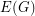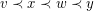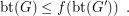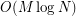# Book Thickness of Subdivisions

 Importance: Medium ✭✭
 Author(s): Blankenship, Robin Oporowski, Bogdan
 Subject: Graph Theory
 Keywords: book embedding book thickness
 Posted by: David Wood on: January 19th, 2009

Letbe a finite undirected simple graph.

A-page book embedding ofconsists of a linear orderofand a (non-proper)-colouring ofsuch that edges with the same colour do not cross with respect to. That is, iffor some edges, thenandreceive distinct colours.

One can think that the vertices are placed along the spine of a book, and the edges are drawn without crossings on the pages of the book.

The book thickness of, denoted by btis the minimum integerfor which there is a-page book embedding of.

Letbe the graph obtained by subdividing each edge ofexactly once.

Conjecture   There is a functionsuch that for every graph,The conjecture is due to [B099]. The conjecture is true for complete graphs [BO99,EM99,E02]. The conjecture is discussed in depth in [DW05].

## Bibliography

*[BO99] Robin Blankenship and Bogdan Oporowski. Drawing Subdivisions Of Complete And Complete Bipartite Graphs On Books, Technical Report 1999-4, Department of Mathematics, Louisiana State University, 1999.

[DW05] Vida Dujmovic and David Wood. Stacks, queues and tracks: Layouts of graph subdivisions. Discrete Mathematics & Theoretical Computer Science 7:155-202, 2005.

[EM99] Hikoe Enomoto and Miki Shimabara Miyauchi. Embedding graphs into a three page book withcrossings of edges over the spine. SIAM J. Discrete Math., 12(3):337–341, 1999.

[E02] David Eppstein. Separating thickness from geometric thickness. In Proc. 10th International Symp. on Graph Drawing (GD ’02), pp. 150–161. vol. 2528 of Lecture Notes in Comput. Sci. Springer, 2002.

* indicates original appearance(s) of problem.

### This problem is solved

This problem is solved in:

V. Dujmović, D. Eppstein, R. Hickingbotham, P. Morin, D. R. Wood. Stack-number is not bounded by queue-number. Combinatorica, accepted in 2021 https://arxiv.org/abs/2011.04195